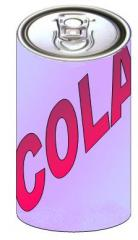An aluminium can contains 330 ml of cola.

If the can’s diameter is 6 cm what is the can’s height?

Suppose the can’s height is 10 cm, what does the diameter have to be?

Which of these two cans uses the least aluminium?

If you could choose any diameter which dimensions for the can would use the least amount of aluminium to hold 330 ml of cola?